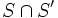Subgroup series

Definition

Symbol-free definition

A subgroup series in a group is an order-preserving map from a totally ordered indexing set to the collection of subgroups of the group, ordered by inclusion.

Definition with symbols

A subgroup series in a group$G$ is a map from a totally ordered indexing set$W$ to the collection of subgroups of$G$, that sends$w$ in$W$ to$H_w$, such that if$v < w$, then$H_v$ is a subgroup of$H_w$.

Particular cases

Well-ordered indexing set (for minimum)

When the indexing set is well-ordered, the subgroup series is termed an ascending series. For any well-ordered indexing set, every element is either a successor element or a limit element. If, further, we have the property that for a limit element, the corresponding subgroup is the union of the subgroups below it, we call the ascending series limit-tight.

Well-ordered indexing set (for maximum)

When every subset has a maximum (that is, the indexing set is well-ordered with respect to maximum), the subgroup series is termed a descending series. If, further, we have the property that for a limit element, the corresponding subgroup is the intersection of the subgroups above it, we call the descending series limit-tight.

Operations on subgroup series

Concatenation of subgroup series

Consider a subgroup series$S_1$ in a group$G$ where the intersection of all members of the series is$H$. Let$S_2$ be a subgroup series in$H$. Then the concatenation of$S_2$ with$S_1$ is a subgroup series of$G$ which essentialyl concatenates the subgroup series. PLACEHOLDER FOR INFORMATION TO BE FILLED IN: [SHOW MORE]

Intersection of subgroup series

Let$S$ and$S'$ be two subgroup series in a group$G$ both indexed by the same indexing set$V$. Then, the intersection of$S$ and$S'$, denoted$S \cap S'$, associated to each element$v \in V$ the subgroup$S_v \cap S_v'$.

Join of subgroup series

Let$S$ and$S'$ be two subgroup series in a group$G$ both indexed by the same indexing set$V$. Then, the intersection of$S$ and$S'$, denoted$S \cap S'$, associated to each element$v \in V$ the subgroup$$.

Maps from subgroup properties to subgroup series properties

The successor condition map

Given a subgroup property$p$, the successor condition map gives a subgroup series property that a subgroup series satisfies if and only if:

• For any member$H_w$,$H_w$ satisfies$p$ as a subgroup of the intersection of all$H_v$ for$v > w$.
• For any member$H_w$, the subgroup generated by$H_v$ for$v < w$ satisfies the property$p$ as a subgroup of$H_w$.

The comparison condition map

Given a subgroup property$p$, the comparison condition map gives the property of being a subgroup series where, given any subsets$U$ and$V$ of$W$ such that every element of$U$ is less than every element of$V$ the subgroup generated by all the subgroups corresponding to$U$ satisfies$p$ as a subgroup of the intersection of all the subgroups corresponding to$V$.